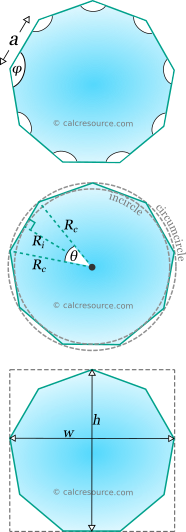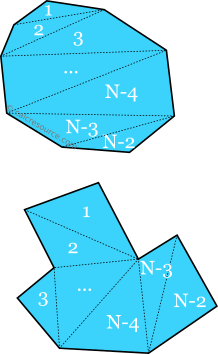## Regular n-gon calculator

This tool calculates the basic geometric properties of a regular n-gon, that is a polygon having n sides and n vertices. Regular polygons are equilateral (all sides equal) and also equiangular (all interior angles equal). The tool can calculate the properties of any regular n-gon, given either the edge length, the inradius, the circumradius, the area, the height or the width. Enter below the shape dimensions. The calculated results will have the same units as your input. Please use consistent units for all input.

 Known data: Side length Inradius Circumradius Area Height Width N = Geometric properties: Area = Perimeter = Side α = Ri = Rc = Bounding box: Height h = Width w = Angles : deg rad Interior φ = Central θ =## Theoretical background

### Definitions

N-gon is a polygon with N sides and N vertices. An n-gon, can be either convex or concave, as illustrated in the next figure. A convex polygon has none of its interior angles greater than 180°. To the contrary, a concave polygon has one or more of its interior angles greater than 180°. A polygon is called regular when its sides are equal and also its interior angles are equal. Having only the sides equal is not adequate to guarantee that the interior angles are also equal. As demonstrated in the figure below, many possible polygons can be defined, with equal sides, but unequal interior angles. These polygons are called equilateral. A special case of equilateral polygons is the so called star polygons. Any n-gon that is not regular is called irregular.

The simplest non-degenerate n-gon is the triangle with N=3. N-gons with N<3 are degenerate. The digon with N=2 has two vertices and two edges. The two edges coincide in the plane, resulting in a shape that looks like a linear segment between the two vertices. The monogon, with N=1, has one vertex and one side that connects the vertex with itself. The following table presents the established naming convention for polygons, depending on the number of edges N:

Any n-gon with N>3, can be constructed as an assembly of triangles. In fact the number of triangles required to construct an n-gon is always the same and equal to N-2. Since the sum of interior angles in a triangle is constant to 180° (or ), the sum of the interior angles in an n-gon, either convex or concave is also constant and equal to: .A polygon with N vertices can be constructed by a minimum of N-2 triangles

### Properties of regular n-gons

The focus comes to the regular polygons, hereafter. A regular polygon has all its edges equal and all its interior angles equal.

#### Symmetry

A regular n-gon features N axes of symmetry. All these axes meet at a common point, the center of the n-gon. If N is an even number, half of the axes pass through diagonally opposite vertices and the remaining ones, pass through the midpoints of opposite edges. On the other hand, if N is odd, all the axes of symmetry, pass through a vertex and the midpoint of its opposite edge.

#### Interior angle and central angle

A regular n-gon has equal interior angles by definition. Since there are N of them, and given that their total sum is , as explained earlier, it can be concluded that each interior angle should be equal to:

From the last expression, it is seen that the interior angle should be lower than radians (or 180°). In other words the regular n-gon cannot be concave. The value of , reaches asymptotically , increasing the number of N.

The central angle , is the angle inside the triangle that is highlighted in the figure below. Specifically, it is the angle between two triangle edges, connecting the center of the n-gon with two successive vertices. The third edge in an edge of the n-gon. There are N central angles, in total, around the common center, therefore each one should be:

The remaining two angles, in the highlighted triangle, are equal to (a line passing through the n-gon center and a vertex is a symmetry axis).

It is worthy to mention, that the interior and central angles are supplementary, since their sum is :

#### Circumcircle and incircle

In any regular n-gon, a circle can be drawn that passes through all vertices. This is the cirmuscribed circle or circumcircle. The center of this circle is the center of the n-gon. The radius of circumcircle is usually called circumradius.

Another circle can also be drawn, that passes through the midpoints of the n-gon edges. This circle is called inscribed circle or incircle. The radius of incircle is usually called inradius. The incircle is tangent to all N edges and its center is the same with that of the circumcircle.

The radii of circumcircle, , and incircle, , are related to the length of the edges, . These relationships can be discovered using the right triangle, with sides: the circumradius, the inradius and half the n-gon edge, which is shown in the next figure. Using basic trigonometry we may find:

where the central angle and the side length. Substituting the equation for the central angle into the last expressions become:

.

The above formulas reveal that for increasing values of N, the inradius asymptotically tends to the circumradius (because approaches zero, and the cosine of the third equation approaches unity). It can be visualized that for large N's, the polygon looks very circular in shape, and therefore its circumcircle and incircle match more perfectly with the n-gon shape and as a result with each other.

#### Area

The total area of a regular n-gon can be divided into N identical isosceles triangles, as indicated in the figure below. The height of any of these triangles, perpendicular to the n-gon edge , is indeed a radius of the incircle, therefore its length is equal to . Therefore, the area of each triangle is , and as a result, the total area of the N triangles becomes:

Alternatively, using the relationship , the n-gon area can be expressed in terms of the circumradius, , this way:

To reach to the last formula, the trigonometric identity was utilized, as well the relationship between and , which, as seen before, is and in inverted form: .

#### Perimeter

The perimeter of any N-sided regular polygon is simply the sum of the lengths of all edges:

.

### Bounding box

The bounding box of a planar shape is the smallest rectangle that encloses the shape completely. The bounding box is defined by its height and width . For a regular n-gon, the width is parallel to an edge while the height is perpendicular to it. Universal formulas for these dimensions are not possible though. A methodology can be improvised however, based on the value of N.

##### Height

Depending on N, the height, , can be equal to:

• the distance between two opposite edge midpoints, if N is even
• the distance between a vertex and the midpoint of an opposite edge, if N is odd.

In either case the height crosses the n-gon center. Therefore:

##### Width

Depending on N, the width, , can be equal to either:

• the distance between two opposite edge midpoints, or
• the distance between two opposite vertices.

If N is an even number, the width passes through the center of the polygon. This does not happen though, if N is an odd number. Therefore, two cases should be examined:

N is even

In this case, the width passes through the center of the polygon. If N/2 is even too, then the width connects two opposite vertices. If N/2 is odd on the other hand the width connects the midpoints of two opposite edges. In other words:

N is odd

In this case, the width connects two opposite vertices, in other words it is a diagonal of the n-gon. Since N is odd, N-1 should be even, by default. Depending on the parity of (N-1)/2 (odd or even) though, the diagonal that defines width can be either above the center of the n-gon or below the it. Specifically:

• if (N-1)/2 is even, the diagonal of lies above the center
• if (N-1)/2 is odd, the diagonal of lies below the center

The next figure illustrates these two sub-cases. The calculation of also becomes straightforward if the angle , in the highlighted triangle, is found. This can be done, by counting the number of central angles until we reach the vertex of required diagonal, starting from the top. It is:

Substituting we get the same result for both sub-cases:

The hypotenuse of the highlighted triangle is equal to the circumradius while the opposite. The edge opposite to to angle , is half the wanted width. Therefore, if the number of the regular n-gon edges is odd, the width can be calculated with the formula:

### Examples

##### Example 1

Determine the circumradius, the inradius and the area of a regular 17-gon, with edge length equal to.

The circumradius and the inradius, in terms of the edge length , for the regular n-gon, have been derived in the previous sections. These are:

Therefore, we have to simply substitute and . Doing so we get:

The angle is approximately equal to: .

Similarly, the inradius is calculated this way:

Finally, the area of a regular n-gon is given by the following equation:

Substituting and we get:

##### Example 2

Calculate the edge length of the following regular n-gons:

1. a 9-gon (aka nonagon or enneagon), with area
2. a 12-gon (aka dodecagon) having height
3. a 4-gon (aka square) having circumradius
##### 1. Regular 9-gon with given area

The area of a regular n-gon, , in terms of the side length , is given by the equation:

Since we are looking for , we have to rearrange the formula:

From the last equation we can calculate the required edge length , if we substitute and for the 9-gon:

.

##### 2. Regular 12-gon with given height

Because N=12, which is an even number, the height , of the 12-gon is equal to:

The inradius, , is given be the formula:

Therefore:

.

##### 3. Regular 4-gon with given circumradius

The circumradius, , of a regular n-gon is related to its edge length, , with the following formula:

Therefore:

From the last equation we can calculate the wanted edge length , if we substitute and , for the given 4-gon:

.

##### Example 3

Find the edge of a regular, hexagon having the same area with a regular 15-gon, with edge length

The area of a regular n-gon is given by the formula:

Applying this formula specifically, for the hexagon and the 15-gon we get:

It is , which means:

Rearranging:

Substituting , to the last equation, we get the wanted edge length, of the regular hexagon:

### Regular n-gon cheat-sheet

In the following table a concise list of the main formulas, related to the regular n-gon is included.

### Table of regular n-gon properties

In the following table, some key properties of various n-gons are presented. Included are the interior angle , the central angle and the ratios, , , , and where: